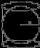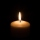# Division + cylinder - math problems

#### Number of problems found: 8

• Diameter of a cylinderThe diameter of the cylinder is 42 cm. How many times does the cylinder turn on a 66m long track?
• Equilateral cylinderA sphere is inserted into the rotating equilateral cylinder (touching the bases and the shell). Prove that the cylinder has both a volume and a surface half larger than an inscribed sphere.
• LatheCalculate the percentage of waste if the cube with 53 cm long edge is lathed to cylinder with a maximum volume.
• PoolThe pool has the shape of a cylinder with a diameter 4.6m, the presumed height is 130cm. The bucket fits 12l of water. How many buckets do you have to bring to fill this pool?
• LatheFrom the cube of edge, 37 cm was a lathed maximum cylinder. What percentage of the cube is left as waste after lathed?
• Wax candlesA company makes wax candles in the shape of a cylinder. Each candle has a diameter of 4 inches and a height of 7 inches. If the company used 7561.12 in3 of wax, how many candles did it make? Use 3.14, and do not round your answer
• WinchThe steel rope has a diameter of 6mm and a length of 20m. We are winding on drum width 60mm, starting diameter 50mm. What is the final diameter after winding?
• Two bodiesThe rectangle with dimensions 8 cm and 4 cm is rotated 360º first around the longer side to form the first body. Then, we similarly rotate the rectangle around the shorter side b to form a second body. Determine the ratio of surfaces of the first and seco

We apologize, but in this category are not a lot of examples.
Do you have an interesting mathematical word problem that you can't solve it? Submit a math problem, and we can try to solve it.

We will send a solution to your e-mail address. Solved examples are also published here. Please enter the e-mail correctly and check whether you don't have a full mailbox.

Please do not submit problems from current active competitions such as Mathematical Olympiad, correspondence seminars etc...

Division Problems. Cylinder Problems.